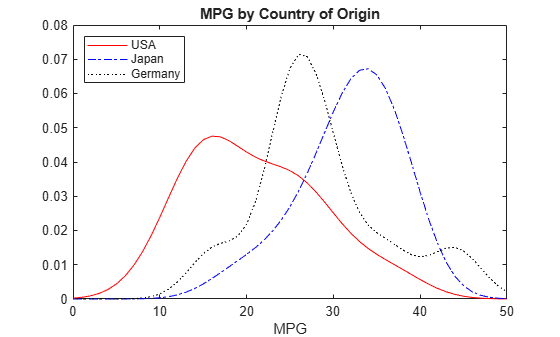# Fit Probability Distribution Objects to Grouped Data

This example shows how to fit probability distribution objects to grouped sample data, and create a plot to visually compare the pdf of each group.

### Step 1. Load sample data.

`load carsmall;`

The data contains miles per gallon (`MPG`) measurements for different makes and models of cars, grouped by country of origin (`Origin`), model year (`Model_Year`), and other vehicle characteristics.

### Step 2. Create a categorical array.

Transform `Origin` into a categorical array.

`Origin = categorical(cellstr(Origin));`

### Step 3. Fit kernel distributions to each group.

Use `fitdist` to fit kernel distributions to each country of origin group in the `MPG` data.

`[KerByOrig,Country] = fitdist(MPG,'Kernel','by',Origin)`
```KerByOrig=1×6 cell array {1x1 prob.KernelDistribution} {1x1 prob.KernelDistribution} {1x1 prob.KernelDistribution} {1x1 prob.KernelDistribution} {1x1 prob.KernelDistribution} {1x1 prob.KernelDistribution} ```
```Country = 6x1 cell {'France' } {'Germany'} {'Italy' } {'Japan' } {'Sweden' } {'USA' } ```

The cell array `KerByOrig` contains six kernel distribution objects, one for each country represented in the sample data. Each object contains properties that hold information about the data, the distribution, and the parameters. The array `Country` lists the country of origin for each group in the same order as the distribution objects are stored in `KerByOrig`.

### Step 4. Compute the pdf for each group.

Extract the probability distribution objects for Germany, Japan, and USA. Use the positions of each country in `KerByOrig` shown in Step 3, which indicates that Germany is the second country, Japan is the fourth country, and USA is the sixth country. Compute the pdf for each group.

```Germany = KerByOrig{2}; Japan = KerByOrig{4}; USA = KerByOrig{6}; x = 0:1:50; USA_pdf = pdf(USA,x); Japan_pdf = pdf(Japan,x); Germany_pdf = pdf(Germany,x);```

### Step 5. Plot the pdf for each group.

Plot the pdf for each group on the same figure.

```plot(x,USA_pdf,'r-') hold on plot(x,Japan_pdf,'b-.') plot(x,Germany_pdf,'k:') legend({'USA','Japan','Germany'},'Location','NW') title('MPG by Country of Origin') xlabel('MPG')```The resulting plot shows how miles per gallon (`MPG`) performance differs by country of origin (`Origin`). Using this data, the USA has the widest distribution, and its peak is at the lowest `MPG` value of the three origins. Japan has the most regular distribution with a slightly heavier left tail, and its peak is at the highest `MPG` value of the three origins. The peak for Germany is between the USA and Japan, and the second bump near 44 miles per gallon suggests that there might be multiple modes in the data.